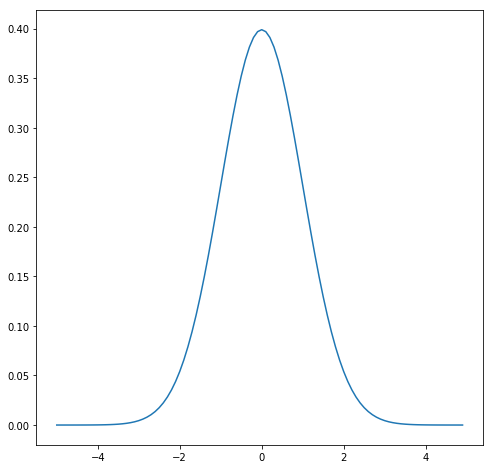## The Normal Distribution

Diving deeper into data science I started to brush up my knowledge about math especially statistics.

## The Mother of all DistributionsThe normal distribution was formulated by Carl Friedrich Gauß in 18XX and can be implemented in Python like the following :

```def normal_distribution(x, mu=0, sigma=1):
sqrt_two_pi = math.sqrt(2*math.pi)
return math.exp(-(x-mu)**2 / 2 / sigma**2) / sqrt_two_pi * sigma```